# Carboxylic acids and Derivatives – Organic Chemistry – list of solved problems

Go to the page with the solutions to the problems.

### Problem 1)

Rank in increasing order of acidity the following series of carboxylic acids.

• a) HCOOH, CH3-CH2-COOH, CH3-COOH
• b) CH3-COOH, CCl3-COOH, CHCl2-COOH, CH2Cl-COOH
• c) CH3-COOH, CH2F-COOH, CH2Br-COOH, CH2Cl-COOH
• d) CH3-CH2-COOH, CH2Cl-CH2-COOH, CH3-CHCl-COOH

### Problem 2)

Represent the resonant structures of the conjugate base of acetic acid.

### Problem 3)

Indicate the product obtained by treating acetic acid with the following reagents:

• a) Ammonia
• b) Thionyl chloride
• d) Hot aniline
• e) Phosphorus pentoxide
• f) Isopropanol in acid medium

### Problem 4)

Describe in detail the mechanism of ester formation in acid medium, and indicate some procedure to promote the shift of equilibrium towards ester formation.

### Problem 5)

Indicate the result of treating propyl pentanoate with an excess of methanol in acid medium.

### Problem 6)

Describe the result of treating benzoyl chloride with 4-aminobutan-1-ol.

### Problem 7)

Represent the scheme of the reaction that takes place when acetic anhydride is treated with p-chloroaniline.

### Problem 8)

Assuming you have three bottles of chlorobenzene, chlorobutane, and benzoyl chloride whose labels have been misplaced, how would you know which one is which by some simple analytical test?

### Problem 9)

Indicate what is the result of reacting salicylic acid (2-hydroxybenzoic acid) with acetic anhydride.

### Problem 10)

What is the result of reacting succinic anhydride with ethylamine?

### Problem 11)

Propose a method for the synthesis of N-methylbutanamide from an ester.

### Problem 12)

Draw the structure of the compounds obtained in the acid, HCl, (dilute) and basic, NaOH, (dilute) hydrolysis of a) ethyl acetate, MeCOOEt and b) methyl propanoate, EtCOOMe.

### Problem 13)

What products are obtained in the following reaction?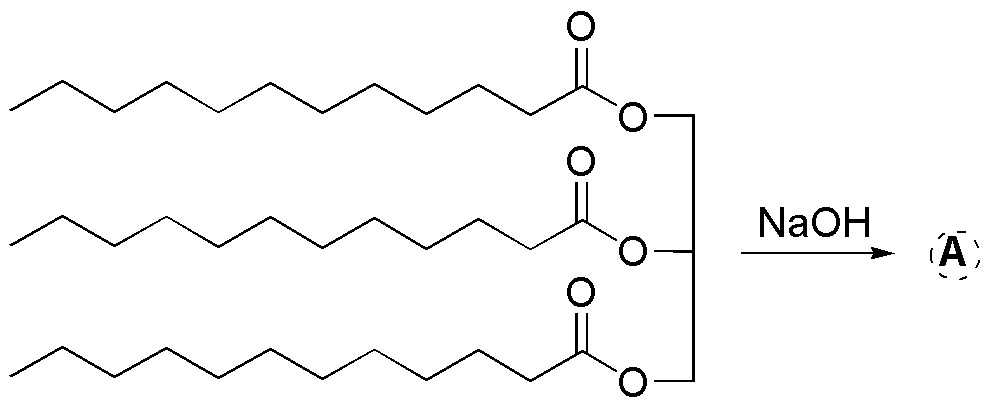### Problem 14)

Complete the following reactions with the major product obtained: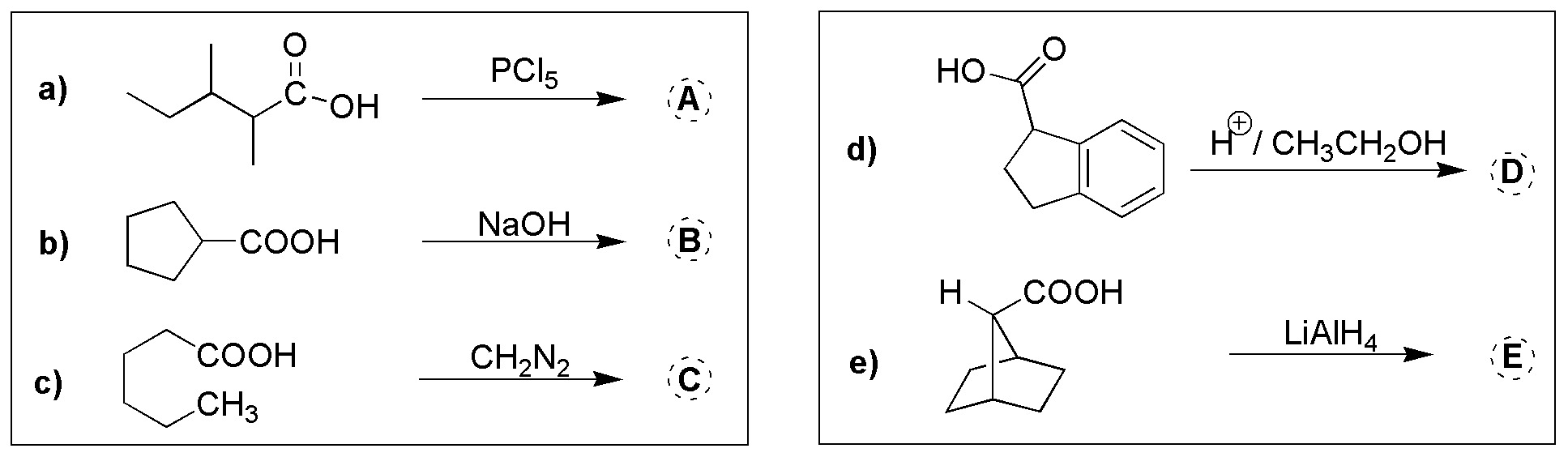### Problem 15)

State the reactants needed to carry out the following reactions (a-e):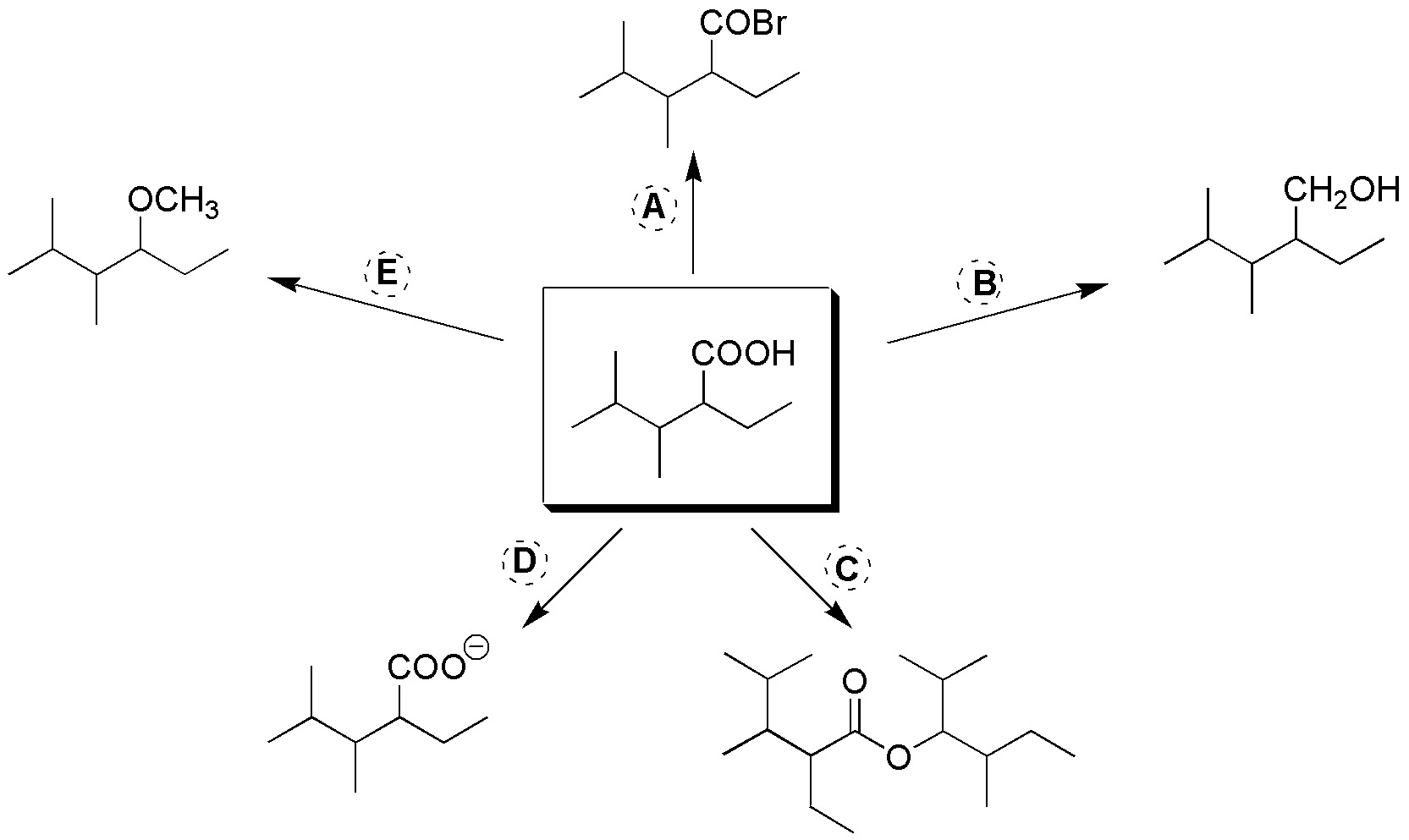### Problem 16)

Complete the following scheme (a-g):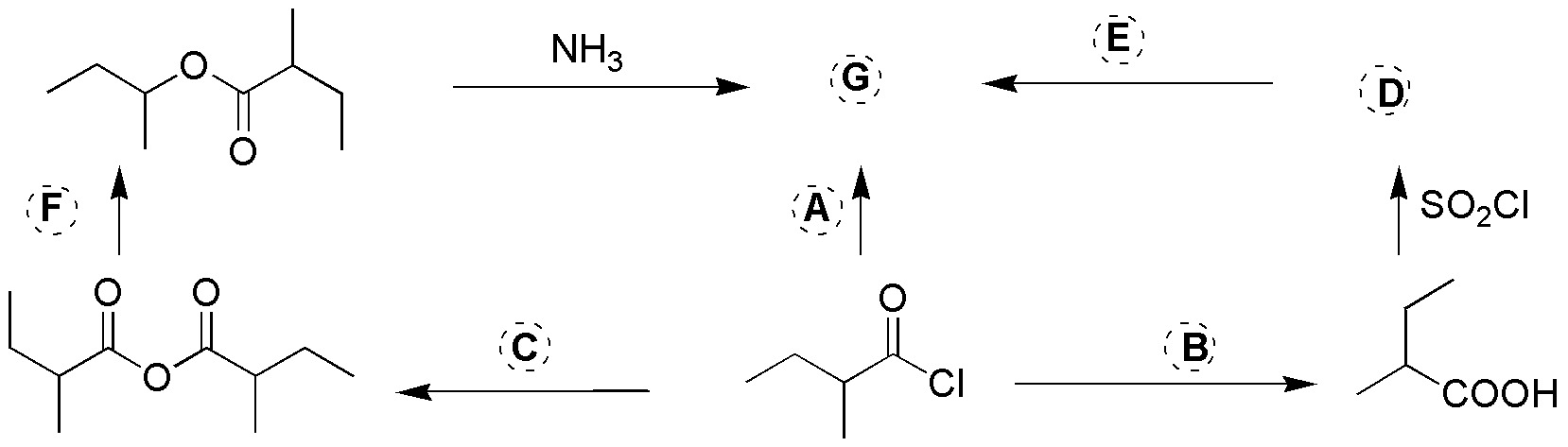### Problem 17)

Complete the following scheme for obtaining phthalimide.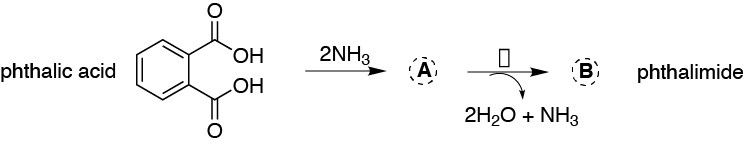### Problem 18)

Complete the major product of the following reactions (a-e):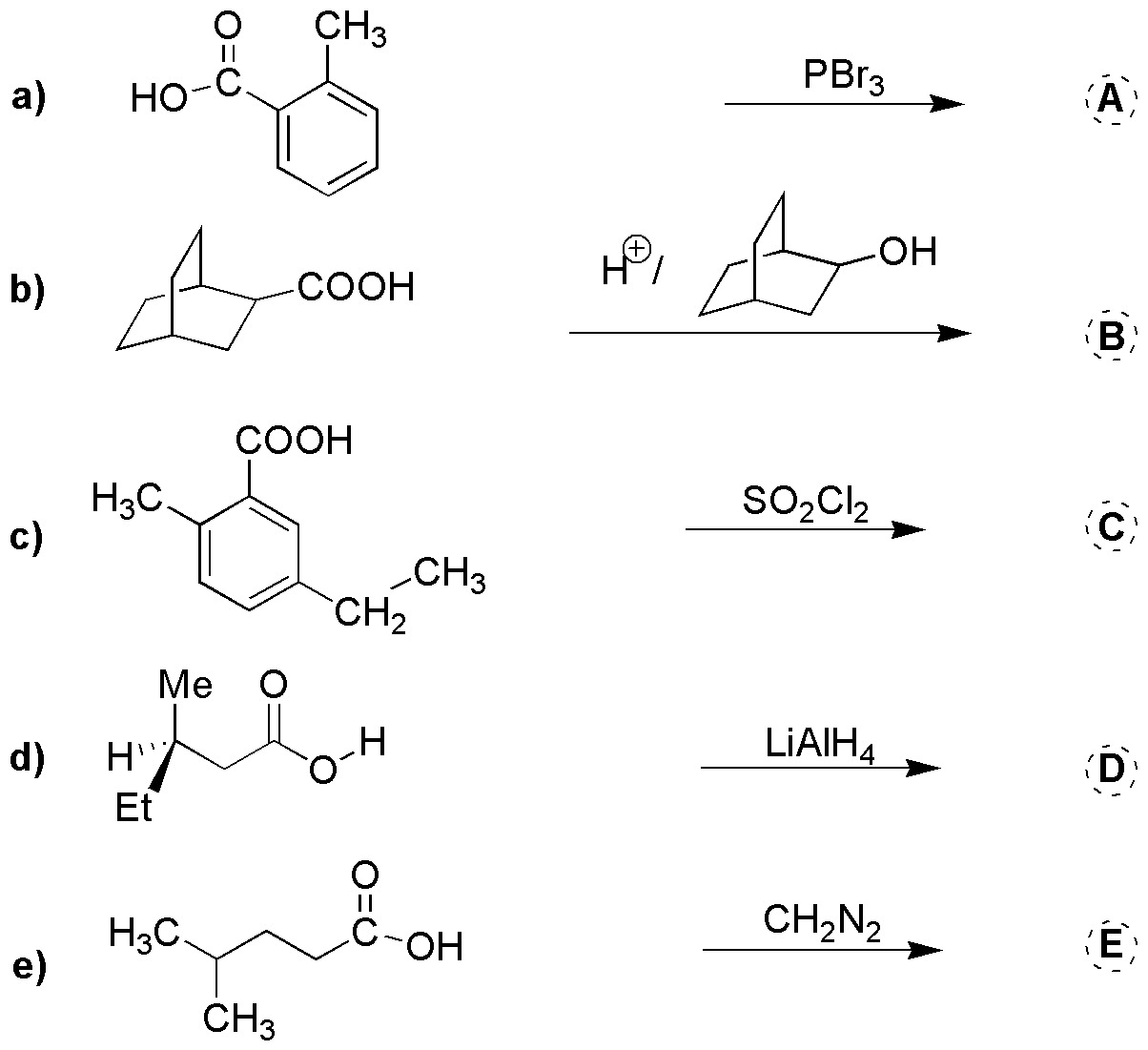### Problem 19)

Indicate the reactants necessary to carry out the following reactions (a-e):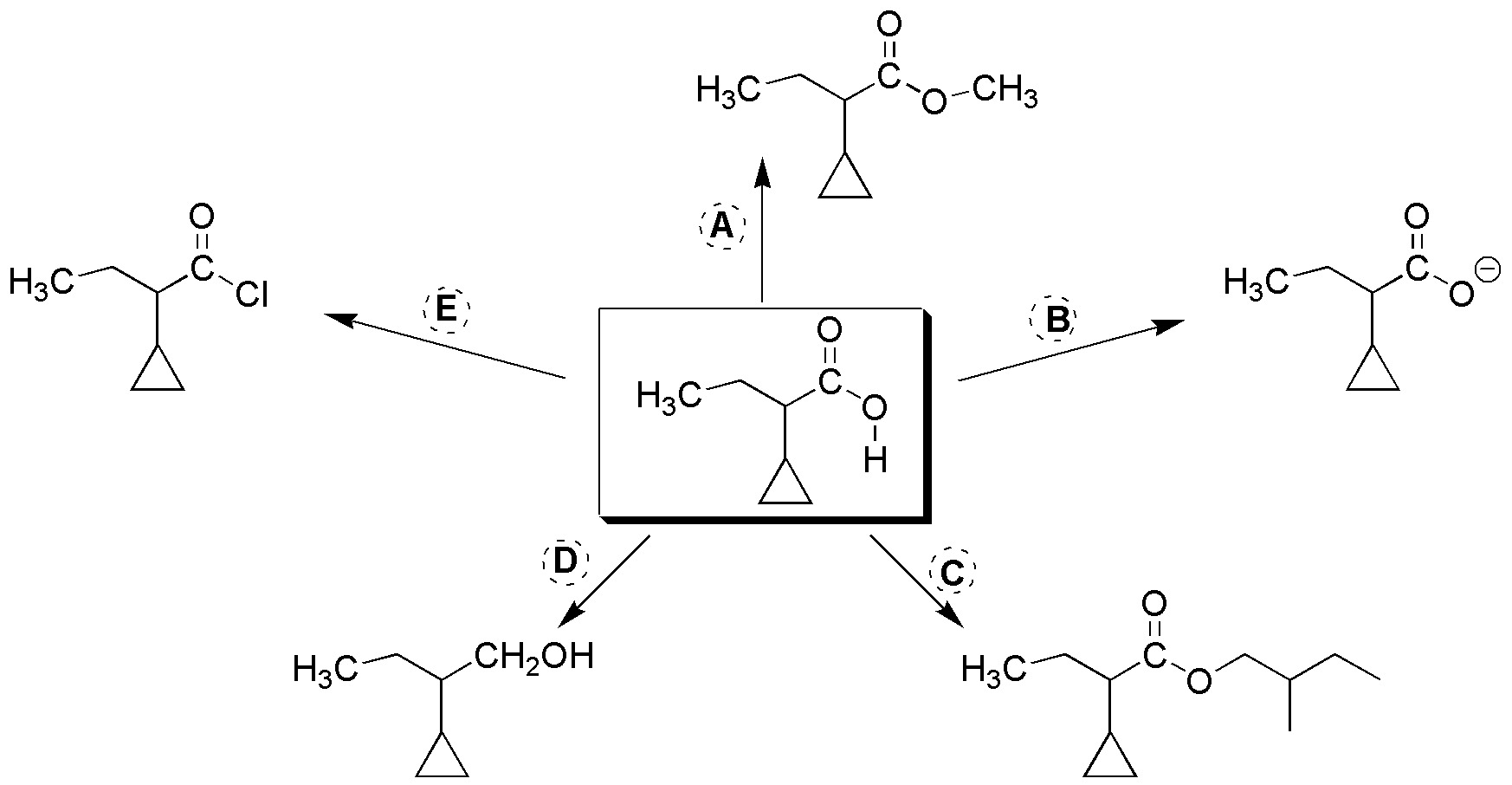### Problem 20)

Complete the following scheme (a-e):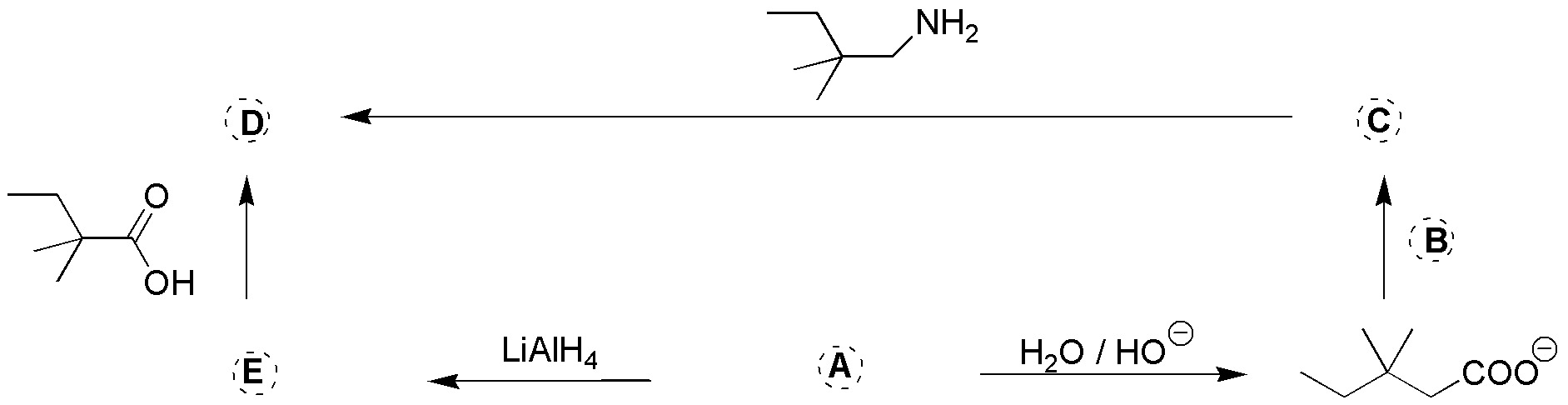### Problem 21)

Complete the following scheme (a-g):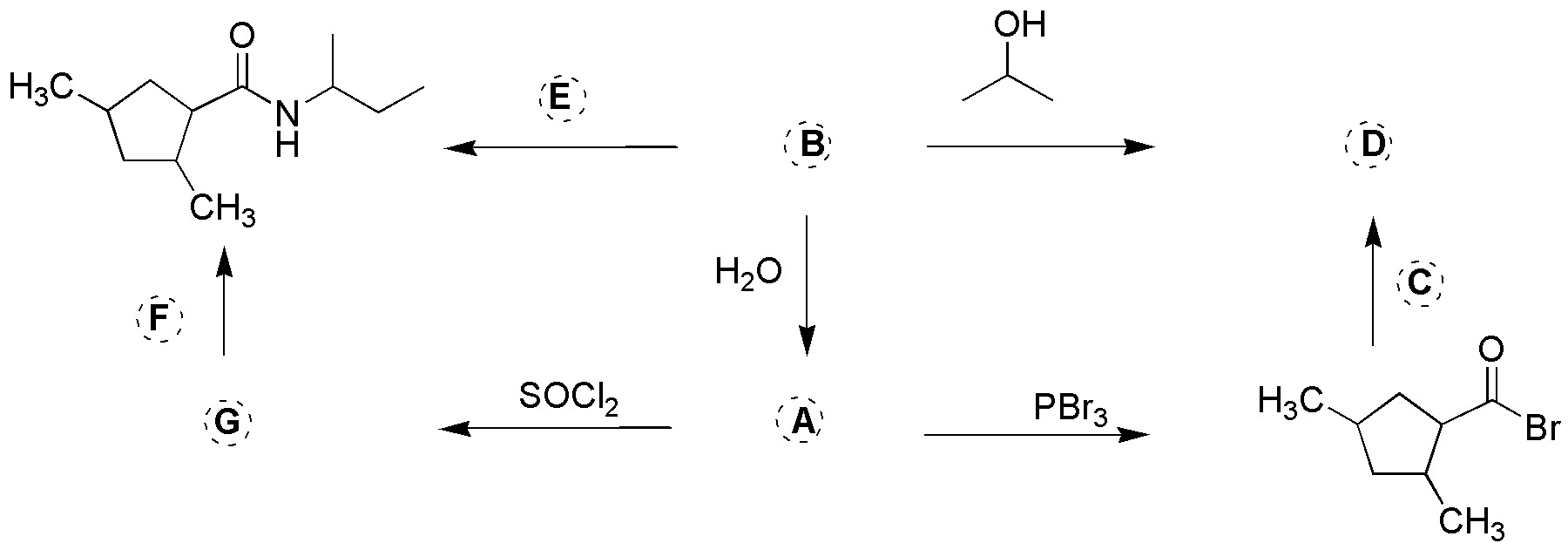### Problem 22)

Complete the following scheme (a-e):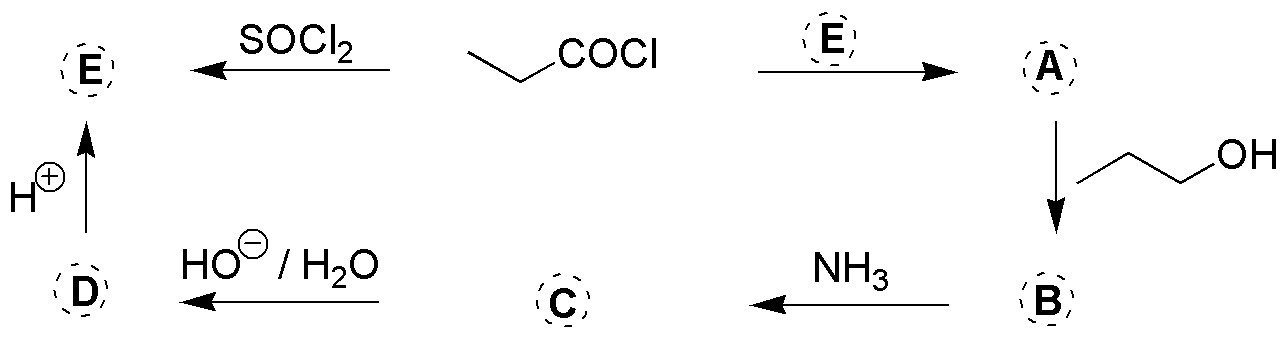### Problem 23)

Complete the following scheme (a-d):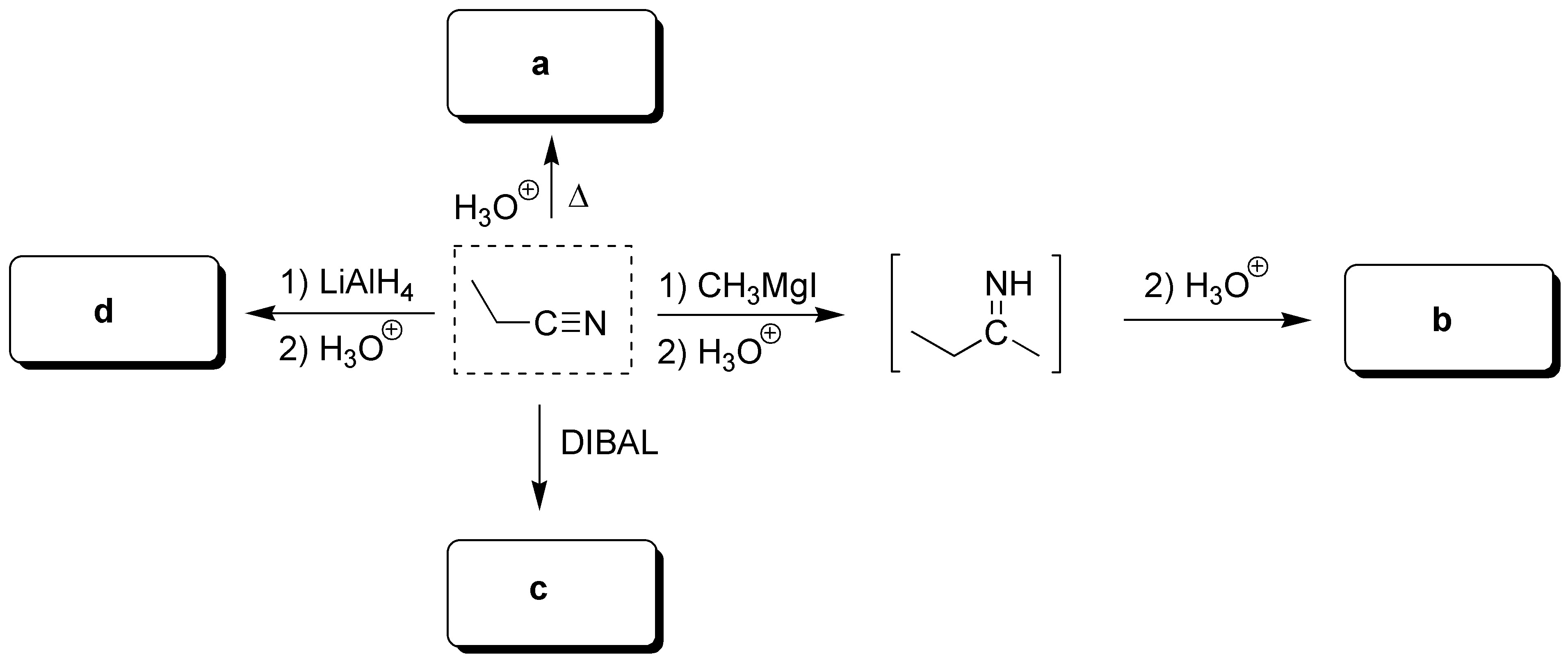### Problem 24)

Complete the following reactions: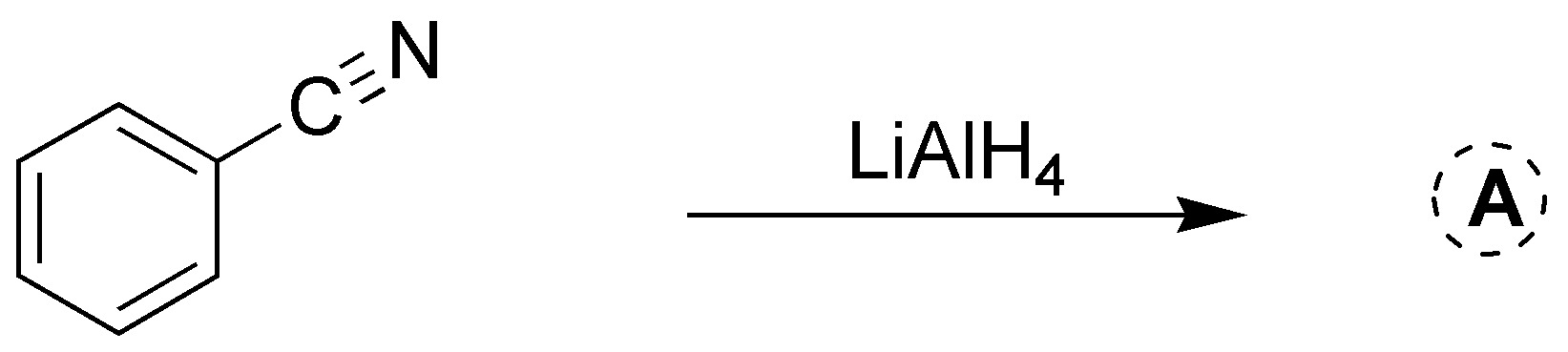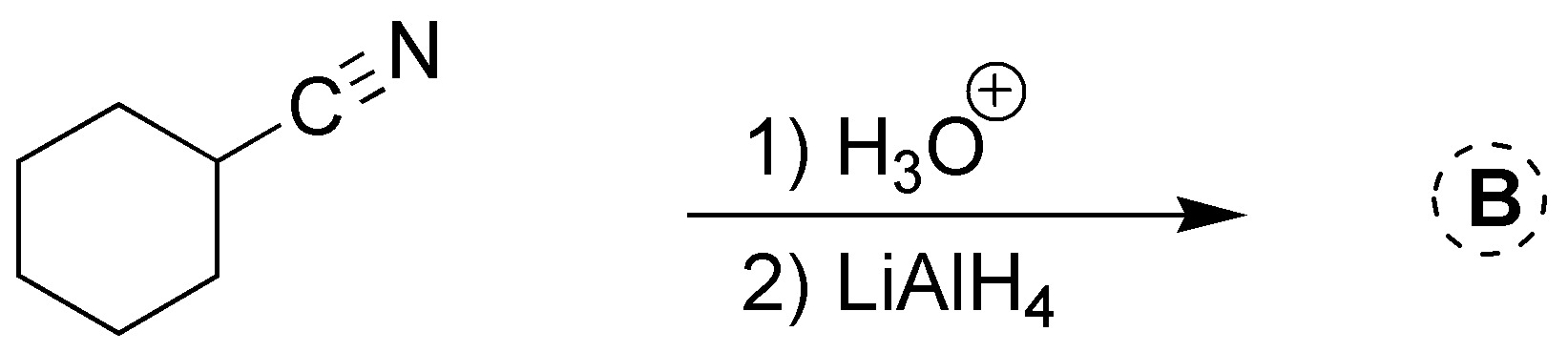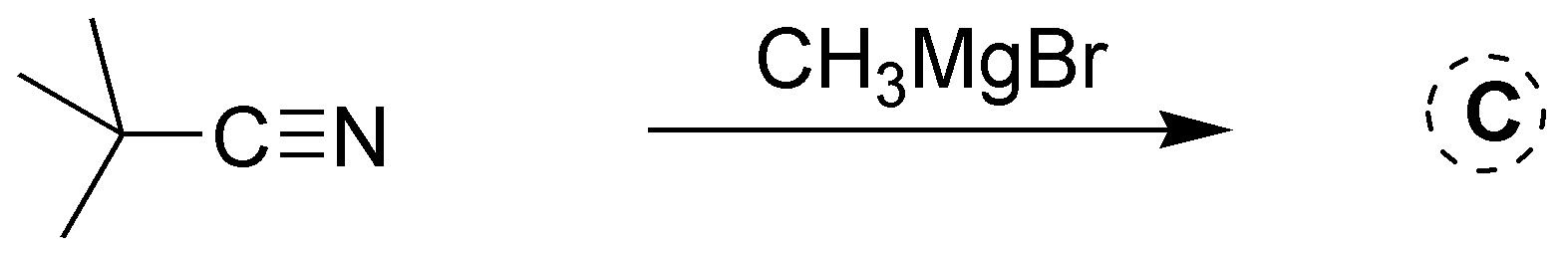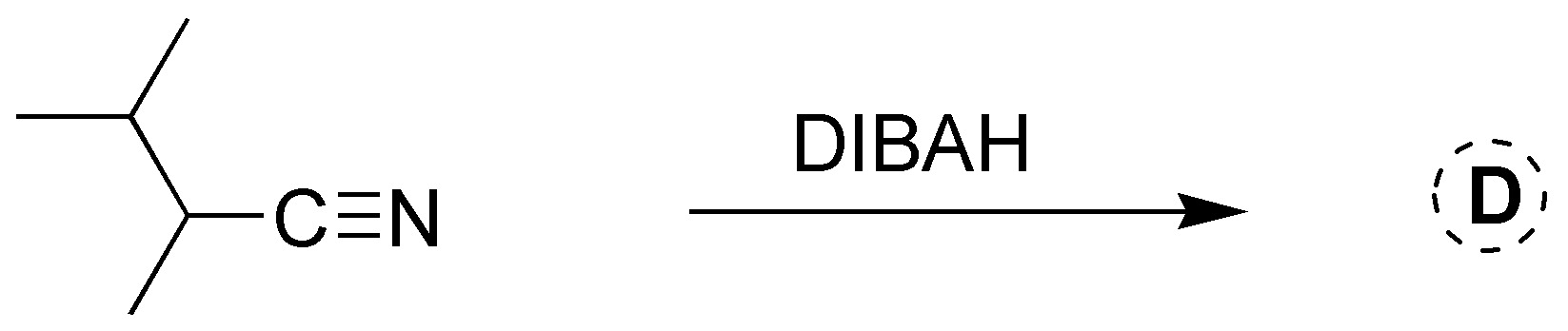### Problem 25)

Propose a mechanism for the following reaction: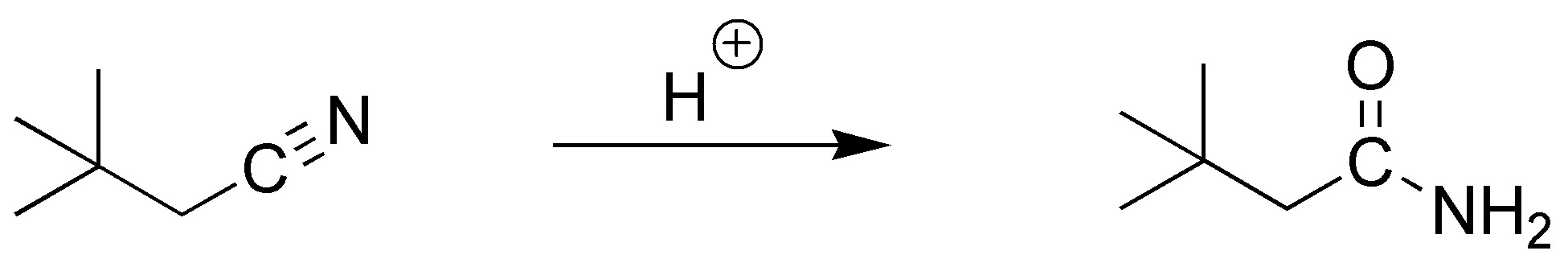### Problem 26)

Choose the appropriate nitrile to obtain the following compounds, and indicate the procedure to follow: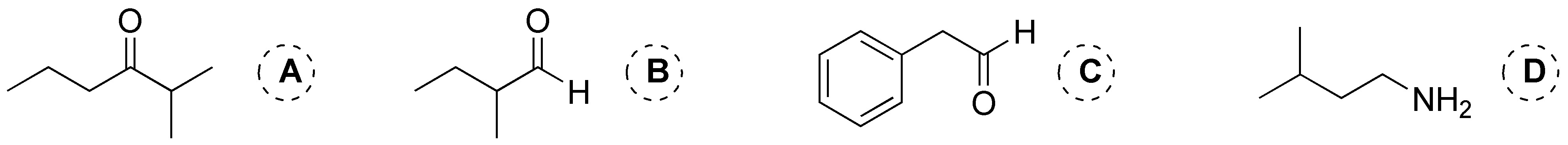Shares